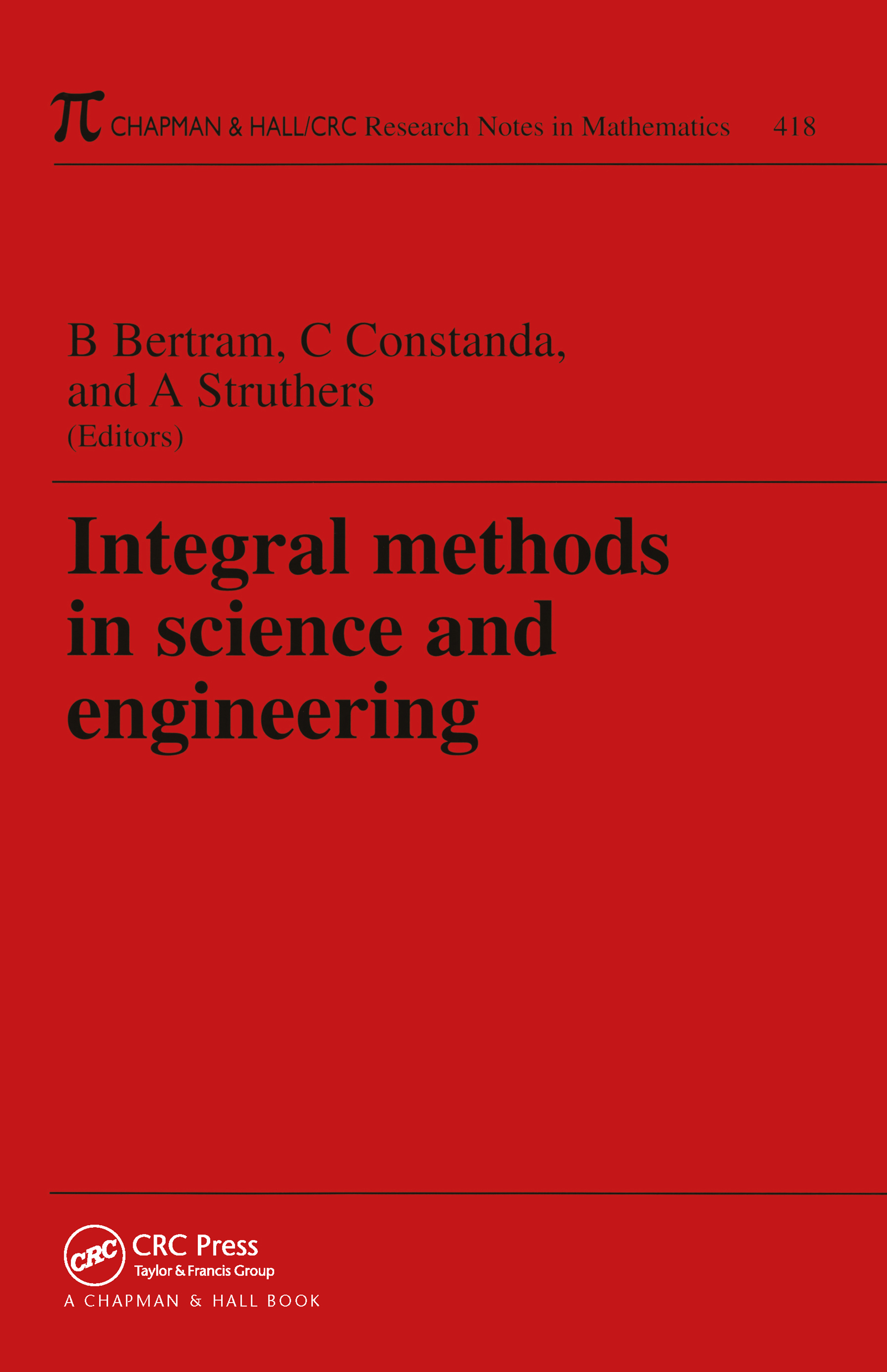# Integral Methods in Science and Engineering

## 1st Edition

Chapman and Hall/CRC

376 pages

Paperback: 9781584881469
pub: 2000-03-23
Currently out of stock
\$215.00
x
eBook (VitalSource) : 9780429123634
pub: 2019-05-20
from \$102.50

FREE Standard Shipping!

### Description

The Proceedings of the Fifth International Conference on Integral Methods in Science and Engineering, form a collection of papers addressing the solution of mathematical problems from various physical domains by integral methods in conjunction with various approximation schemes. Written by acknowledged experts, these peer-reviewed papers offer recent developments in both theory and application valuable to applied mathematicians, engineers, and physicists.

### Reviews

"The book provides inspiration for further research and can be strongly recommended to applied a mathematicians, engineers, and physicists."

Mathematical Modeling of N-Body Quantum Scattering Processes

Microhydrodynamics of Sharp Corners and Edges

Acoustic Scattering by Irregular Obstacles

On Generalized Inverse Steklov Problems

A Hybrid Root Finder

Volterra Type Integral Geometry Problems

Inversionof the X-Ray Transform and the Radon Transform with Incomplete Data

Reconstructing a Function by means of Integrals over a Family of Conical Surfaces

On the use of Wavelet Expansions and the Conjugate Gradient Method for Solving First Kind Integral Equations

Optimal Algorithms for the Calculation of Singular Integrals

An Order One Approximate Method of Solution of Differential and Integral Equations

A Multigrid Method for Solving Reaction-Diffusion Systems

Time-Dependent Bending of Plates with Transverse Shear Deformation

Polarization Gradient in Piezoelectric Micropolar Elasticity

Thermoelastic Stress Separation via Poisson Equation Solution by means of the Boundary Element Method

On Neutral Functional Differential Equations with Causal Operations

A Semi-Analytic Method for the Study of Acoustic Pulse Propagation in Inhomogeneous Elastic 1-D Media

Efficient Finite Elements for the Numerical Approximation of Cylindrical Shells

Error Estimates for Computing Fixed Densities of Markov Integral Operators

A Modified Monte Carlo Approach to the Approximation of Invariant Measures

Acceleration Waves in von Kármán Plate Theory

Dynamics and Resonance of a Nonlinear Mechanical Oscillator Subjected to Parametric and External Excitation

On the Vibration of Helical Springs

A Two-Dimensional Numerical Model of Chemotaxis

Asymptotic Analysis of Fracture Theory for Layered Composites in Compression (The Plane Problem)

Existence and Regularity of Weak Solutions to the Displacement Boundary Value Problem of Nonlinear Elastostatics

On Convergence and Uniqueness of Microscale Heat Transfer Equation

On Numerical Approximations of a Frictionless Contact Problem for Elastic-Viscoplastic Materials

A Panel Clustering Method for 3-D Elastostatics using Spherical Harmonics

Extensions of Constrained Least-Squares for Obtaining Regularized Solutions to First-Kind Integral Equations

Existence and Nonexistence Results for some Boundary Value Problems at Resonance

Analytic Investigation of Thick Anisotropic Plates with Undulating Surfaces

On Stoke's Nonlinear Integral Wave Equation

Dynamics of the Spurt Phenomenon for Single History Integral Constitutive Equations

A Boundary Integral Equation Method for the Heat Equation

Computational Simulation and Interfacial Shear Models for Downward Annular Wavy-Interface Condensing Flow in a Vertical Pipe

Compact Fourth-Order Approximation for a Nonlinear Reaction-Diffusion Equation arising in Population Genetics

Dynamic Deformation of a Layered Continuum Surrounding a Cylindrical or Spherical Cavity

Boundary Value Problems for Harmonic Vector Fields on Non-Smooth Domains

The Oblique Derivative Problem for General Elliptic Systems in Lipschitz Domains

Viscous Aerodynamic Optimal Design of Flying Configurations via an Enlarged Variational Method

Interface Crack Problem for a Piecewise Homogeneous Anisotropic Plane

Micromechanics of Heterogeneous Materials

Admissibility and Optimal Control for Stochastic Difference Equations

Linear and Sublinear Tricomi via DFI

Multidimensional Fractional Integrals on Spaces of Smooth Functions

Piecewise Polynomial Projection Methods for Nonlinear, Multidimensional, Weakly Singular Integral Equations

Asymptotics and Inequalities for a Class of Infinite Sums

A New Theorem Concerning the Buckingham-Rayleigh Methods and General Dimensional Analysis

Energy Decay for a Weak Solution of the Non-Newtonian Fluid Equations with Slowly Varying External Forces

An Integral Representation for the Solution of 2-D Non-Stationary Flow of Micropolar Fluids

A Monotonically Convergent Adaptive Method for Nonlinear Combustion Problems

Stationary Oscillations of Elastic Plates with Robin Boundary Conditions

Adapter and Driver Design for Rotary Encoders

Expert System Design for Fault Diagnosing in CNC Machine Tools

Non-Ideal Liquid Solutions Modeling by means of Integral Methods

Symmetry Groups, Conservation Laws, and Group-Invariant Solutions of the Marguerre-von Kármán Equations

Modeling the Motion of an Underwater Explosion Bubble

On some New Systems of N-ary Integral Equations

Computational Simulations and Flow Domain Classification for Laminar/Laminar annular/Stratified Condensing Flows